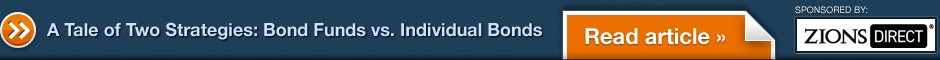## Bank Loan Bond Funds

### Categories:

BlackRock
BlackRock Floating Rate Income A (BFRAX)
BlackRock Floating Rate Income C (BFRCX)
BlackRock Floating Rate Income I (BFRIX)
Columbia
Columbia Floating Rate A (RFRAX)
Columbia Floating Rate B (RSFBX)
Columbia Floating Rate C (RFRCX)
Columbia Floating Rate I (RFRIX)
Columbia Floating Rate R (CFRRX)
Columbia Floating Rate R5 (RFRFX)
Columbia Floating Rate W (RFRWX)
Columbia Floating Rate Z (CFRZX)
DWS Investments
DWS Floating Rate Plus A (DFRAX)
DWS Floating Rate Plus C (DFRCX)
DWS Floating Rate Plus Instl (DFRTX)
DWS Floating Rate Plus S (DFRPX)
Eaton Vance
Eaton Vance Floating Rate A (EVBLX)
Eaton Vance Floating Rate Adv (EABLX)
Eaton Vance Floating Rate B (EBBLX)
Eaton Vance Floating Rate C (ECBLX)
Eaton Vance Floating Rate I (EIBLX)
Eaton Vance Floating-Rate & Hi Inc A (EVFHX)
Eaton Vance Floating-Rate & Hi Inc Adv (EAFHX)
Eaton Vance Floating-Rate & Hi Inc B (EBFHX)
Eaton Vance Floating-Rate & Hi Inc C (ECFHX)
Eaton Vance Floating-Rate & Hi Inc Inst (EIFHX)
Eaton Vance Floating-Rate Advantage A (EAFAX)
Eaton Vance Floating-Rate Advantage B (EBFAX)
Eaton Vance Floating-Rate Advantage C (ECFAX)
Eaton Vance Floating-Rate Advantage I (EIFAX)
Fidelity Investments
Fidelity Advisor Float Rate Hi Inc A (FFRAX)
Fidelity Advisor Float Rate Hi Inc B (FFRBX)
Fidelity Advisor Float Rate Hi Inc C (FFRCX)
Fidelity Advisor Float Rate Hi Inc I (FFRIX)
Fidelity Advisor Float Rate Hi Inc T (FFRTX)
Fidelity Floating Rate High Income (FFRHX)
Hartford Mutual Funds
Hartford Floating Rate A (HFLAX)
Hartford Floating Rate B (HFLBX)
Hartford Floating Rate C (HFLCX)
Hartford Floating Rate I (HFLIX)
Hartford Floating Rate R3 (HFLRX)
Hartford Floating Rate R4 (HFLSX)
Hartford Floating Rate R5 (HFLTX)
Hartford Floating Rate Y (HFLYX)
Highland Funds
Highland Floating Rate A (XLFAX)
Highland Floating Rate Advantage A (XSFRX)
Highland Floating Rate Advantage B (XSFBX)
Highland Floating Rate Advantage C (XLACX)
Highland Floating Rate Advantage Z (XLAZX)
Highland Floating Rate B (XLSBX)
Highland Floating Rate C (XLFCX)
Highland Floating Rate Z (XLFZX)
ING Funds
ING Floating Rate A (IFRAX)
ING Floating Rate C (IFRCX)
ING Floating Rate I (IFRIX)
ING Floating Rate R (IFRRX)
ING Floating Rate W (IFRWX)
ING Senior Income A (XSIAX)
ING Senior Income B (XSIBX)
ING Senior Income C (XSICX)
ING Senior Income I (XSIIX)
ING Senior Income W (XSIWX)
Invesco
Invesco Floating Rate A (AFRAX)
Invesco Floating Rate C (AFRCX)
Invesco Floating Rate I (AFRIX)
Invesco Floating Rate R (AFRRX)
Invesco Floating Rate Y (AFRYX)
Invesco Prime Income Trust (XPITX)
Invesco Van Kampen Sr Loan A (VSLAX)
Invesco Van Kampen Sr Loan B (VSLBX)
Invesco Van Kampen Sr Loan C (VSLCX)
Invesco Van Kampen Sr Loan IB (XPRTX)
Invesco Van Kampen Sr Loan IC (XSLCX)
John Hancock
JHancock2 Floating Rate Income 1 (JFIHX)
JHancock2 Floating Rate Income A (JFIAX)
JHancock2 Floating Rate Income B (JFIBX)
JHancock2 Floating Rate Income C (JFIGX)
JHancock2 Floating Rate Income I (JFIIX)
JHancock2 Floating Rate Income NAV (JFIDX)
Lord Abbett
Lord Abbett Floating Rate A (LFRAX)
Lord Abbett Floating Rate C (LARCX)
Lord Abbett Floating Rate F (LFRFX)
Lord Abbett Floating Rate I (LFRIX)
Lord Abbett Floating Rate R2 (LFRRX)
Lord Abbett Floating Rate R3 (LRRRX)
MainStay
MainStay Floating Rate A (MXFAX)
MainStay Floating Rate B (MXFBX)
MainStay Floating Rate C (MXFCX)
MainStay Floating Rate I (MXFIX)
MainStay Floating Rate Inv (MXFNX)
Neuberger Berman
Neuberger Berman Floating Rate Inc A (NFIAX)
Neuberger Berman Floating Rate Inc C (NFICX)
Neuberger Berman Floating Rate Inc Inst (NFIIX)
Pioneer Investments
Pioneer Floating Rate A (FLARX)
Pioneer Floating Rate C (FLRCX)
Pioneer Floating Rate Y (FLYRX)
Putnam
Putnam Floating Rate Income A (PFLRX)
Putnam Floating Rate Income B (PFRBX)
Putnam Floating Rate Income C (PFICX)
Putnam Floating Rate Income Y (PFRYX)
RidgeWorth
RidgeWorth Seix Floating RT High Inc A (SFRAX)
RidgeWorth Seix Floating RT High Inc C (SFRCX)
RidgeWorth Seix Floating RT High Inc I (SAMBX)
RS Funds
RS Floating Rate A (RSFLX)
RS Floating Rate C (RSFCX)
RS Floating Rate K (RSFKX)
RS Floating Rate Y (RSFYX)
SunAmerica
SunAmerica Senior Floating Rate A (SASFX)
SunAmerica Senior Floating Rate C (NFRCX)
T. Rowe Price
T. Rowe Price Instl Floating Rate (RPIFX)
Virtus
Virtus Senior Floating Rate A (PSFRX)
Virtus Senior Floating Rate C (PFSRX)
Virtus Senior Floating Rate I (PSFIX)